NEET  >  Test: Structure of Atom - 3

# Test: Structure of Atom - 3

Test Description

## 30 Questions MCQ Test Chemistry Class 11 | Test: Structure of Atom - 3

Test: Structure of Atom - 3 for NEET 2023 is part of Chemistry Class 11 preparation. The Test: Structure of Atom - 3 questions and answers have been prepared according to the NEET exam syllabus.The Test: Structure of Atom - 3 MCQs are made for NEET 2023 Exam. Find important definitions, questions, notes, meanings, examples, exercises, MCQs and online tests for Test: Structure of Atom - 3 below.
Solutions of Test: Structure of Atom - 3 questions in English are available as part of our Chemistry Class 11 for NEET & Test: Structure of Atom - 3 solutions in Hindi for Chemistry Class 11 course. Download more important topics, notes, lectures and mock test series for NEET Exam by signing up for free. Attempt Test: Structure of Atom - 3 | 30 questions in 60 minutes | Mock test for NEET preparation | Free important questions MCQ to study Chemistry Class 11 for NEET Exam | Download free PDF with solutions
 1 Crore+ students have signed up on EduRev. Have you?
Test: Structure of Atom - 3 - Question 1

### Radiation of λ = 155 nm was irradiated on Li (work function = 5eV) plate. The stopping potential (in eV) is.

Detailed Solution for Test: Structure of Atom - 3 - Question 1

3 eV
Corite Actual
Correct option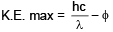= 8 – 5
= 3eV
∴ V0 = 3 eV

Test: Structure of Atom - 3 - Question 2

### Increasing order of magnetic moment among the following species is __________ . Na+, Fe+3, Co2+, Cr+2

Detailed Solution for Test: Structure of Atom - 3 - Question 2

Na+, Co+2, Cr2+, Fe+3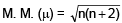we get Na+, Co+2, Cr2+, Fe+3

Test: Structure of Atom - 3 - Question 3

### If in the hydrogen atom P.E. at ∞ is choosen to be 13.6 eV then the ratio of T.E. to K.E. for 1st orbit of H-atom is __________ .

Detailed Solution for Test: Structure of Atom - 3 - Question 3

Zero
Refrence level is Ist orbit
itself T. E. = 0
Ratio becomes zero

Test: Structure of Atom - 3 - Question 4

The light radiations with discrete quantities of energy are called ___________ .

Detailed Solution for Test: Structure of Atom - 3 - Question 4

A photon is the quantum of electromagnetic radiation. The term quantum is the smallest elemental unit of a quantity, or the smallest discrete amount of something. Thus, one quantum of electromagnetic energy is called a photon. The plural of quantum is quanta.

Test: Structure of Atom - 3 - Question 5

The ratio of the energy of a photon of 2000 Å wavelength radiation to that of 4000 Å radiation is

Detailed Solution for Test: Structure of Atom - 3 - Question 5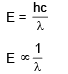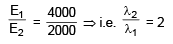Test: Structure of Atom - 3 - Question 6

The energy of electron is maximum at :

Detailed Solution for Test: Structure of Atom - 3 - Question 6

An electron bound to the nucleus by the mutual attraction between unlike charges, has a negative energy. Its most tightly bound to the nucleus nearer it is to the nucleus. That is an electron has least energy when it is in the n=1 shell. As the electron moves away from the nucleus its energy becomes less and less negative, i.e. its energy increases. When the electron is not under the attractive Coulomb force of the nucleus its potential energy is zero and whatever energy it has now, is kinetic energy, which is always positive. So farther the electron more energy it has.

Test: Structure of Atom - 3 - Question 7

Which electronic level would allow the hydrogen atom to absorb a photon but not to emit a photon:

Detailed Solution for Test: Structure of Atom - 3 - Question 7

Is → As it is the ground state

Test: Structure of Atom - 3 - Question 8

The third line in Balmer series corresponds to an electronic transition between which Bohr's orbits in hydrogen :

Detailed Solution for Test: Structure of Atom - 3 - Question 8

Balmer means transition to n = 2
1. line → 3 to 2
2. line → 4 to 2
3. line → 5 to 2

Test: Structure of Atom - 3 - Question 9

The orbital angular momentum of an electron in 2s orbital is :

Detailed Solution for Test: Structure of Atom - 3 - Question 9

Orbital Angular momentum is given by [√l(l+1)]×h/2π
For s orbital, we have l = 0
Therefore orbital angular momentum = 0

Test: Structure of Atom - 3 - Question 10

Which quantum number is not related with Schrodinger equation :

Detailed Solution for Test: Structure of Atom - 3 - Question 10

Spin quantum number do not follow Schrodinger Wave Equation because it is related to spin of the electrons.It is needed to explain Pauli's exclusion principle which sates that no two electrons can share the same state (i.e. have the same quantum numbers) at the same time.Therefore, if the quantum numbers n, l and are same then the electrons should have different spin.

Test: Structure of Atom - 3 - Question 11

The shortest wavelength of He atom in Balmer series is x, then longest wavelength in the Paschene series of Li+2 is :

Detailed Solution for Test: Structure of Atom - 3 - Question 11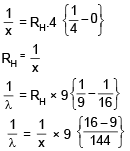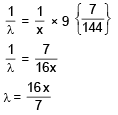Test: Structure of Atom - 3 - Question 12

An electron in a hydrogen atom in its ground state absrobs energy equal to the ionisation energy of Li+2. The wavelength of the emitted electron is

Detailed Solution for Test: Structure of Atom - 3 - Question 12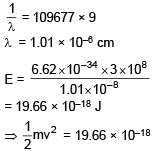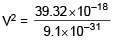V2 = 4.32 × 1013
V2 = 43.2 × 1012
V = 6.57 × 106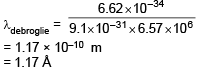Test: Structure of Atom - 3 - Question 13

An electron, a proton and an alpha particle have kinetic energies of 16E, 4E and E respectively. What is the qualitative order of their de-Broglie wavelengths?

Detailed Solution for Test: Structure of Atom - 3 - Question 13

Mass of a particle = 4 (mass of proton)
Mass of proton = 1840 (mass of e)
Let Mass of e = m
∴ Mass of p+ = 1840 m
and mass of a particle = 7360 m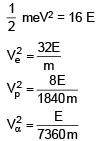Test: Structure of Atom - 3 - Question 14

Given ΔH for the process Li(g) → Li+3(g) + 3e- is 19800 kJ/mole & IE1 for Li is 520 then IE2 & IE3 of Li+ are respectively (approx, value) :

Detailed Solution for Test: Structure of Atom - 3 - Question 14

IE1 + IE2 + IE3 = 19800
IE2 + IE3 = 19800 – 520
IE2 + IE3 = 19280

Test: Structure of Atom - 3 - Question 15

The ratio of difference in wavelengths of 1st and 2nd lines of Lyman series in H-like atom to difference in wavelength for 2nd and 3rd lines of same series is :

Detailed Solution for Test: Structure of Atom - 3 - Question 15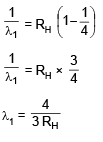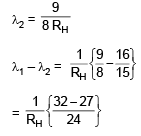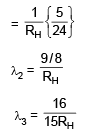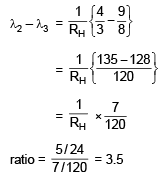Test: Structure of Atom - 3 - Question 16

If radius of second stationary orbit (in Bohr's atom) is R. Then radius of third orbit will be

Detailed Solution for Test: Structure of Atom - 3 - Question 16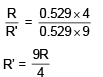= 2.25 R

Test: Structure of Atom - 3 - Question 17

The ratio of wave length of photon corresponding to the α-line of lyman series in H-atom and β-line of Baimer series in He+ is

Detailed Solution for Test: Structure of Atom - 3 - Question 17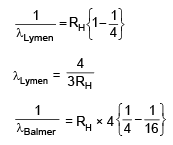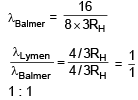Test: Structure of Atom - 3 - Question 18

Three energy levels P, Q, R of a certain atom are such that EP < EQ < ER. If λ1, λ2, and λ3, are the wave length of radiation corresponding to transition R → Q : Q → P and R → P respectively. The correct relationship between λ1, λ2 and λ3 is

Detailed Solution for Test: Structure of Atom - 3 - Question 18

We know that, for wave no.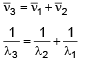Test: Structure of Atom - 3 - Question 19

The Value of (n2 + n1) and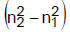for He+ ion in atomic spectrum are 4 and 8 respectively. The wavelength of emitted photon when electron jump from n2 to n1 is

Detailed Solution for Test: Structure of Atom - 3 - Question 19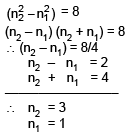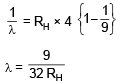Test: Structure of Atom - 3 - Question 20

Number of possible spectral lines which may be emitted in bracket series in H atom if electrons present 9th excited level returns to ground level, are

Detailed Solution for Test: Structure of Atom - 3 - Question 20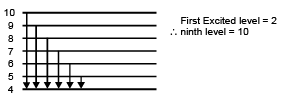Total line = 6

Test: Structure of Atom - 3 - Question 21

The first use of quantum theory to explain the structure of atom was made by :

Detailed Solution for Test: Structure of Atom - 3 - Question 21

Bohr's theory was based upon some postulations of classical physics and some postulations of the newly proposed quantum theory of Planck.

Test: Structure of Atom - 3 - Question 22

The wavelength associated with a golf ball weighing 200g and moving at a speed of 5m/h is of the order:

Detailed Solution for Test: Structure of Atom - 3 - Question 22

From de Broglie wavelength formula; λ = h/p where h=Planck's constant and p=momentum.
Putting all the values, we have h=6.626×10-34/(200/1000)(5/3600)
= 2.3853×10-30
Order is of 10-30

Test: Structure of Atom - 3 - Question 23

The longest wavelength of He+ in Paschen series is "m", then shortest wavelength of Be3+ in Paschen series is (in terms of m) :

Detailed Solution for Test: Structure of Atom - 3 - Question 23

The expression for the wavelength is :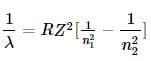For the longest wavelength of the He+ atom in Paschen series , the expression becomes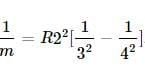(1)

For the shortest wavelength of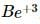the expression becomes(2)
Divide equation (2) with equation (1).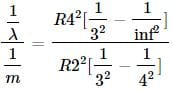On solving this, we get λ=7/64m

Test: Structure of Atom - 3 - Question 24

What is uncertainity in location of a photon of wavelength 5000 Å if wavelength is known to an accuracy of 1 pm?

Detailed Solution for Test: Structure of Atom - 3 - Question 24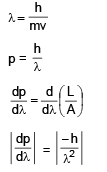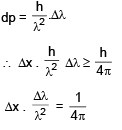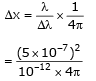0.0199 m

Test: Structure of Atom - 3 - Question 25

Consider the following nuclear reactions involving X & Y.

X → Y + 2He4                      Y → 8O18 + 1H1

If both neutrons as well as protons in both the sides are conserved in nuclear reaction then moles of neutrons in 4.6 gm of X :

Detailed Solution for Test: Structure of Atom - 3 - Question 25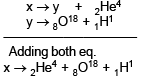By conservation of mass
X = 4 + 18 + 1 gm
= 23
23 gm → (2 + 10) moles neutrons.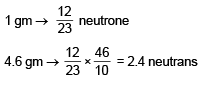Test: Structure of Atom - 3 - Question 26

Electromagnetic radiations having λ = 310 Å are subjected to a metal sheet having work function = 12.8 eV. What will be the velocity of photoelectrons with maximum Kinetic energy.

Detailed Solution for Test: Structure of Atom - 3 - Question 26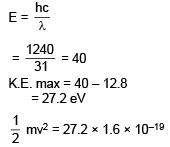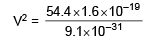V2 = 9.56 × 1012
V = 3.09 × 106 m/sec.

Test: Structure of Atom - 3 - Question 27

Assuming Heisenberg Uncertainity Principle to be true what could be the miniumum uncertainity in de–Broglie wavelength of a moving electron accelerated by Potential Difference of 6V whose uncertainity in position is 7/22 n.m.

Detailed Solution for Test: Structure of Atom - 3 - Question 27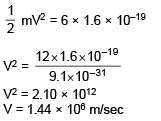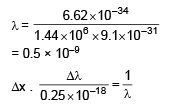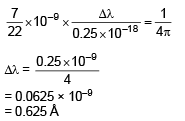Test: Structure of Atom - 3 - Question 28

A 1-kW radio transmitter operates at a frequency of 880 Hz. How many photons per second does it emit -

Detailed Solution for Test: Structure of Atom - 3 - Question 28

Given :
P = 1 kW
P = 1 × 103 watt
E = 103 J/S in one sec
v = 880 Hz
∴ E = nhv
⇒  103 x  ×  6.626 × 10–34 × 880
⇒  x = 1.71 × 1033 in one sec

Test: Structure of Atom - 3 - Question 29

The value of Bohr radius of hydrogen atom is -

Detailed Solution for Test: Structure of Atom - 3 - Question 29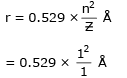= 0.529 × 10–10 m
= 0.529 × 10–8 cm

Test: Structure of Atom - 3 - Question 30

On the basis of Bohr's model, the radius of the 3rd orbit is -

Detailed Solution for Test: Structure of Atom - 3 - Question 30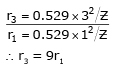## Chemistry Class 11

204 videos|331 docs|229 tests
 Use Code STAYHOME200 and get INR 200 additional OFF Use Coupon Code
Information about Test: Structure of Atom - 3 Page
In this test you can find the Exam questions for Test: Structure of Atom - 3 solved & explained in the simplest way possible. Besides giving Questions and answers for Test: Structure of Atom - 3, EduRev gives you an ample number of Online tests for practice

## Chemistry Class 11

204 videos|331 docs|229 tests

### How to Prepare for NEET

Read our guide to prepare for NEET which is created by Toppers & the best Teachers